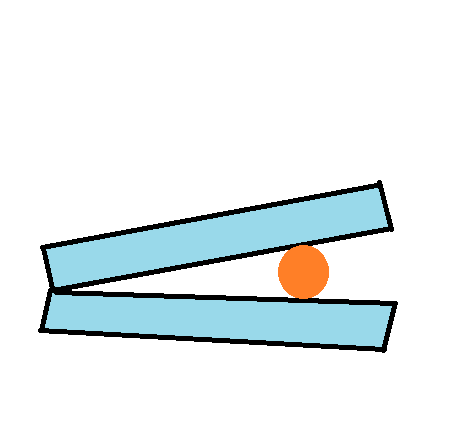The glass plates in the figure are separated by a thin round filament. When the top plate is...

Question:

The glass plates in the figure are separated by a thin round filament. When the top plate is illuminated normally with a light of wavelength 640 nm, the filament lies directly below the tenth order bright fringe. What is the diameter of the filament?Interference of light:

Interference shows the wave nature of light according to which when two light waves which are emitted from the source having the constant phase difference or the source is coherent then light wave superimpose on each other and form alternative bright and dark fringes on the screen.

The wavelength of the light is {eq}\lambda=640\ nm {/eq}

For the bright fringe:

{eq}sin\theta =\frac{n\lambda }{d} {/eq}

For the diameter of the filament:

{eq}d=\frac{n\lambda }{\sin\theta } {/eq}

The top plate is illuminated when the rays fall normally hence angle {eq}\theta=90^\circ {/eq}

Now solving for the filament:

{eq}d=\frac{n\lambda }{\sin\theta }\\ d=\frac{10\times 640}{1}\\ d=6400\ nm {/eq}

Thus, the diameter of the filament is 6400 nm